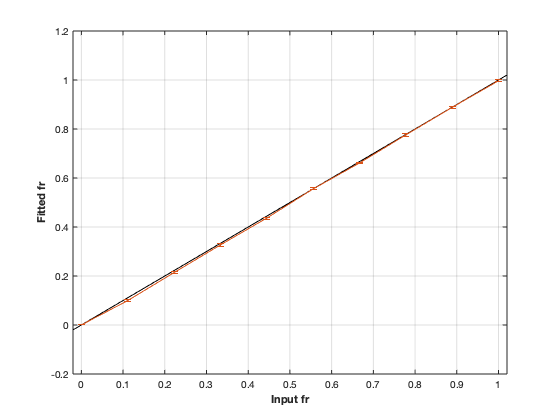# charmed: Composite Hindered and Restricted Model for Diffusion¶## Contents

```% This m-file has been automatically generated using qMRgenBatch(charmed)
% Command Line Interface (CLI) is well-suited for automatization
% purposes and Octave.
%
% Please execute this m-file section by section to get familiar with batch
% processing for charmed on CLI.
%
%
% Written by: Agah Karakuzu, 2017
% =========================================================================
```

## I- DESCRIPTION

```qMRinfo('charmed'); % Describe the model
```
``` charmed: Composite Hindered and Restricted Model for Diffusion
a href="matlab: figure, imshow Diffusion.png ;"Pulse Sequence Diagram/a

Assumptions:
Diffusion gradients are applied perpendicularly to the neuronal fibers.
Neuronal fibers model:
geometry                          cylinders
Orientation dispersion            NO
Permeability                      NO
Diffusion properties:
intra-axonal                      restricted in cylinder with Gaussian
Phase approximation
diffusion coefficient (Dr)       fixed by default. this assumption should have
little impact if the average
propagator is larger than
axonal diameter (sqrt(2*Dr*Delta)8?m).
extra-axonal                      Gaussian
diffusion coefficient (Dh)       Constant by default. Time dependence (lc)

Inputs:
DiffusionData       4D DWI
(SigmaNoise)        map of the standard deviation of the noise per voxel

Outputs:
fr                  Fraction of water in the restricted compartment.
Dh                  Apparent diffusion coefficient of the hindered compartment.
diameter_mean       Mean axonal diameter weighted by the axonal area -- biased toward the larger axons
fixed to 0 -- stick model (recommended if Gmax  300mT/m).
fcsf                Fraction of water in the CSF compartment. (fixed to 0 by default)
lc                  Length of coherence. If  0, this parameter models the time dependence
of the hindered diffusion coefficient Dh.
Els Fieremans et al. Neuroimage 2016.
Interpretation is not perfectly known.
Use option "Time-Dependent Models" to get different interpretations.
(fh)                Fraction of water in the hindered compartment, calculated as: 1 - fr - fcsf
(residue)           Fitting residuals

Protocol:
Various bvalues
diffusion gradient direction perpendicular to the fibers

DiffusionData       Array [NbVol x 7]
Delta (s)         Diffusion separation
delta (s)         Diffusion duration
TE (s)            Echo time

Options:
Rician noise bias               Used if no SigmaNoise map is provided.
'Compute Sigma per voxel'     Sigma is estimated by computing the STD across repeated scans.
'fix sigma'                   Use scd_noise_std_estimation to measure noise level. Use 'value' to fix Sigma.
Display Type
'q-value'                     abscissa for plots: q = gamma.delta.G (?m-1)
'b-value'                     abscissa for plots: b = (2.pi.q)^2.(Delta-delta/3) (s/mm2)
S0 normalization
'Use b=0'                     Use b=0 images. In case of variable TE, your dataset requires a b=0 for each TE.
'Single T2 compartment'       In case of variable TE acquisition:
fit single T2 using data acquired at b1000s/mm2 (assuming Gaussian diffusion))
Time-dependent models
'Burcaw 2015'                 XXX
'Ning MRM 2016'               XXX

Example of command line usage:
Model = charmed;  % Create class from model
Model.Prot.DiffusionData.Mat = txt2mat('Protocol.txt');  % Load protocol
data = struct;  % Create data structure
FitResults = FitData(data,Model,1);  % Fit each voxel within mask
FitResultsSave_nii(FitResults,'DiffusionData.nii.gz');  % Save in local folder: FitResults/

For more examples: a href="matlab: qMRusage(charmed);"qMRusage(charmed)/a

Author: Tanguy Duval, 2016

References:
Please cite the following if you use this module:
Assaf, Y., Basser, P.J., 2005. Composite hindered and restricted model of diffusion (CHARMED) MR imaging of the human brain. Neuroimage 27, 48?58.
In addition to citing the package:
Cabana J-F, Gu Y, Boudreau M, Levesque IR, Atchia Y, Sled JG, Narayanan S, Arnold DL, Pike GB, Cohen-Adad J, Duval T, Vuong M-T and Stikov N. (2016), Quantitative magnetization transfer imaging made easy with qMTLab: Software for data simulation, analysis, and visualization. Concepts Magn. Reson.. doi: 10.1002/cmr.a.21357

Reference page in Doc Center
doc charmed

```

## a- create object

```Model = charmed;
```

## b- modify options

```         |- This section will pop-up the options GUI. Close window to continue.
|- Octave is not GUI compatible. Modify Model.options directly.```
```Model = Custom_OptionsGUI(Model); % You need to close GUI to move on.
```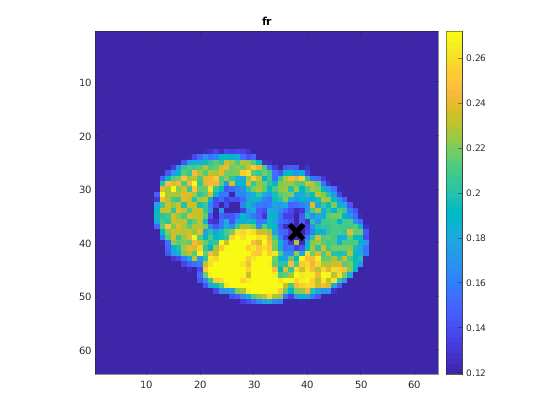## III- FIT EXPERIMENTAL DATASET

```         |- charmed object needs 3 data input(s) to be assigned:
|-   DiffusionData
|-   SigmaNoise
```data = struct();
% DiffusionData.nii.gz contains [64    64     1  1791] data.
% Mask.nii.gz contains [64  64] data.
```

## b- fit dataset

`           |- This section will fit data.`
```FitResults = FitData(data,Model,0);
```
```=============== qMRLab::Fit ======================
Operation has been started: charmed
Elapsed time is 5.586296 seconds.
Operation has been completed: charmed
==================================================
```

## c- show fitting results

```         |- Output map will be displayed.
|- If available, a graph will be displayed to show fitting in a voxel.
|- To make documentation generation and our CI tests faster for this model,
we used a subportion of the data (40X40X40) in our testing environment.
|- Therefore, this example will use FitResults that comes with OSF data for display purposes.
|- Users will get the whole dataset (384X336X224) and the script that uses it for demo
via qMRgenBatch(qsm_sb) command.```
```FitResults_old = load('FitResults/FitResults.mat');
qMRshowOutput(FitResults_old,data,Model);
```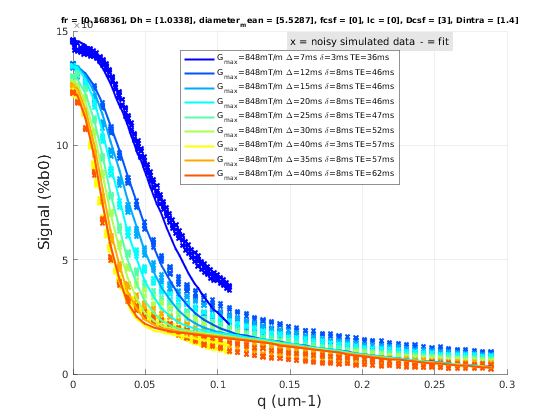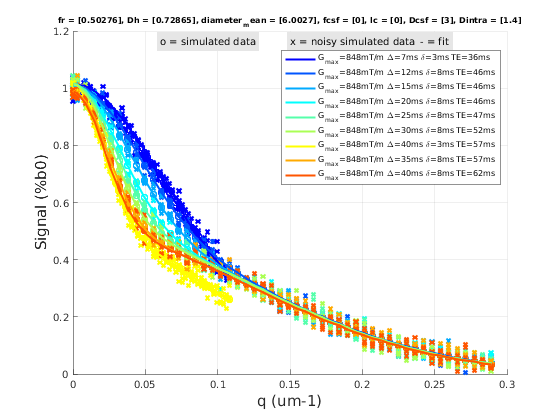## d- Save results

```         |-  qMR maps are saved in NIFTI and in a structure FitResults.mat
that can be loaded in qMRLab graphical user interface
|-  Model object stores all the options and protocol.
It can be easily shared with collaborators to fit their
own data or can be used for simulation.```
```FitResultsSave_nii(FitResults, 'charmed_data/DiffusionData.nii.gz');
Model.saveObj('charmed_Demo.qmrlab.mat');
```
```Warning: Directory already exists.
```

## V- SIMULATIONS

`   |- This section can be executed to run simulations for charmed.`

## a- Single Voxel Curve

```         |- Simulates Single Voxel curves:
(1) use equation to generate synthetic MRI data
(3) fit and plot curve```
```      x = struct;
x.fr = 0.5;
x.Dh = 0.7;
x.diameter_mean = 6;
x.fcsf = 0;
x.lc = 0;
x.Dcsf = 3;
x.Dintra = 1.4;
% Set simulation options
Opt.SNR = 50;
% run simulation
figure('Name','Single Voxel Curve Simulation');
FitResult = Model.Sim_Single_Voxel_Curve(x,Opt);
```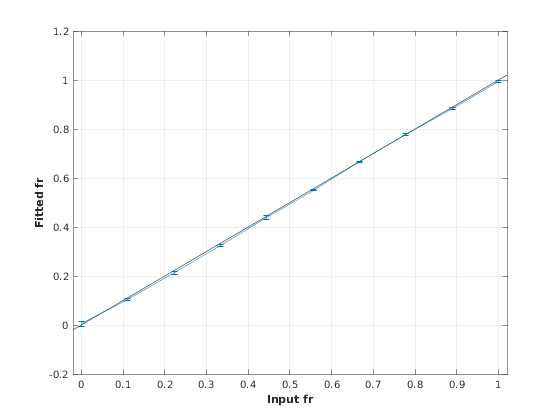## b- Sensitivity Analysis

```         |-    Simulates sensitivity to fitted parameters:
(1) vary fitting parameters from lower (lb) to upper (ub) bound.
(2) run Sim_Single_Voxel_Curve Nofruns times
(3) Compute mean and std across runs```
```      %              fr            Dh            diameter_mean fcsf          lc            Dcsf          Dintra
OptTable.st = [0.5           0.7           6             0             0             3             1.4]; % nominal values
OptTable.fx = [0             1             1             1             1             1             1]; %vary fr...
OptTable.lb = [0             0.3           3             0             0             1             0.3]; %...from 0
OptTable.ub = [1             3             10            1             8             4             3]; %...to 1
% Set simulation options
Opt.SNR = 50;
Opt.Nofrun = 5;
% run simulation
SimResults = Model.Sim_Sensitivity_Analysis(OptTable,Opt);
figure('Name','Sensitivity Analysis');
SimVaryPlot(SimResults, 'fr' ,'fr' );
```